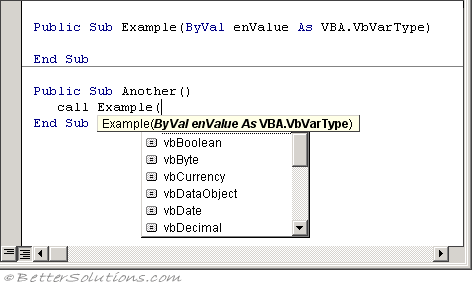### VarType Function

This function returns a number that indicates the data type of the data stored in a variable.
If you use the Variant data type in your code you will eventually be in a situation where you need to know exactly what type of data is stored in this variable.
You can only provide the name of a single variable.
The VarType function returns an enumerated value indicating which data type has been assigned.

`iValue = VarType(variablename) `

where ivariablename is the name of the variable whose sub type you want to find.

#### vbVarType Enumeration

There is an enumeration that you can use to indicate the type of variant object#### Number Returned

There are built-in VBA constants with the same numerical values that you can use instead of the actual numbers.

 Number VBA.vbVarType 8192 (&H2000) vbArray 0 vbEmpty 1 vbNull 2 vbInteger 3 vbLong 4 vbSingle 5 vbDouble 6 vbCurrency 7 vbDate 8 vbString 9 vbOject 10 vbError 11 vbBoolean 12 vbVariant 13 vbDataObject 14 vbDecimal 17 (&H11) vbByte 36 (&H24) vbUserDefinedType

#### Identifying an Array

The number equivalent for vbArray is 8192.
This actual number can never be returned from the VarType Function.
If you pass in an array variable the number that is returned will be greater than 8192.
In fact the number returned is 8192 plus the value of the arrays underlying data type.

`Dim myArray() As Long Call MsgBox(VarType(myArray)) = 8195 `